# Part 5: Vector Proofs | Beginner’s Guide to Year 12 Ext 1 Maths

In this article, we guide you through vector proofs for Maths Extension 1. You can test your knowledge and skills with our concept questions.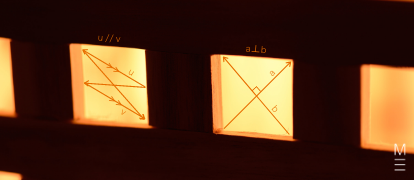In this article, we walk you through how to approach Maths Extension 1 Vector Proofs.

## Year 12 Mathematics Extension 1: Vector Proofs

In this article, we’ll be discussing some vector proofs, both algebraic and geometric, along with more difficult examples. These examples would help consolidate one’s vector knowledge and techniques. In addition, harder examples would assist with vector proofs as mentioned.

Vector proofs involve using all of the vector knowledge being gained, from vector addition to dot products and projections, to prove various algebraic and geometric results. To master vector proofs, one will need a lot of practice and a thorough absorption of knowledge.

### NESA Syllabus Outcomes

• Prove geometric results and construct proofs involving vectors in two dimensions including to proving that:
• the diagonals of a parallelogram meet at right angles if and only if it is a rhombus
• the midpoints of the sides of a quadrilateral join to form a parallelogram
• the sum of the squares of the lengths of the diagonals of a parallelogram is equal to the sum of the squares of the lengths of the sides

## Assumed Knowledge

Students would need to know:

• What a vector is and how they are denoted
• Operations with vector scaling, along with vector addition & subtraction
• Both geometric and algebraic aspects of vectors
• How to split vectors into components
• The dot product and its applications
• Vector projections and how to decompose vectors using them
• Force and velocity as a vector

## Algebraic & Geometric Proofs

It is essential to know that to attempt these algebraic questions, we have a lot of vector properties at our disposal! A few of those important properties include:

• $$cos(θ)= \frac{u⋅v}{|u||v|}$$
• $$u⊥v⇔u⋅v=0$$
• $$u⋅u=|u|^2$$
• $$Proj_v(u)= \left( \frac{u⋅v}{|v|^2} \right) v$$
• Whenever two vectors $$u$$ and $$v$$ are parallel, this mathematically means that $$u$$ is a scalar multiple of $$v$$, or vice versa.
• We can scale a vector $$u$$ down to unit length, i.e. $$\hat u = \frac{u}{|u|}$$, where $$|u|$$ is the magnitude of the vector.

Note: Always remember that to denote a vector running from point A to point B we write it as

$$\overrightarrow{AB} = B-A= target-source$$

With vector proofs, the best way to improve is to familiarise yourself with common questions and techniques used to solve common proofs. In this blog, we will be going through five common proofs questions and the techniques you should use to solve them.

### Example 1:

Prove (using vectors) that the line joining the midpoints of two sides of a triangle is parallel to the third side and half its length.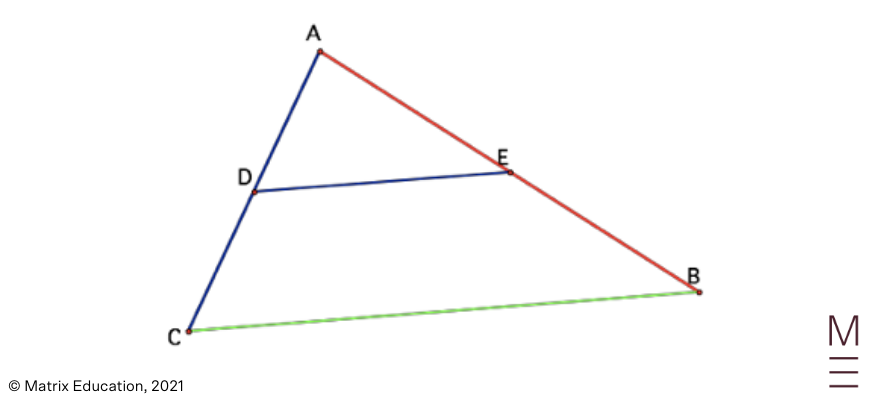### Solution:

Let $$u= \overrightarrow{CD}=\overrightarrow{DA}, v=\overrightarrow{AE}=\overrightarrow{EB}$$. Defining the vectors this way allows for easier to follow calculations.

We are required to prove that $$\overrightarrow{DE} = \frac{1}{2}\overrightarrow{CB}$$.

Hence, we have to rewrite both $$\overrightarrow{DE}$$ and $$\overrightarrow{CB}$$ as $$u$$ and $$v$$. We proceed with vector addition.

Therefore, we can see that

 \begin{align*} \overrightarrow{DE}&=\overrightarrow{DA}+\overrightarrow{AE}\\ &=u+v\\ \end{align*}

and,

 \begin{align*} \overrightarrow{CB}&=\overrightarrow{CA}+\overrightarrow{AB}\\ &=2u+2v\\ &=2(u+v)\\ \end{align*}

Hence,

 \begin{align*} u+v &= \frac{1}{2} \times 2(u+v)\\ ⇒ \overrightarrow{DE} &= \frac{1}{2} \overrightarrow{CB} \ \ \ \text{, as required.}\\ \end{align*}

Example 2:

$$OABC$$ is a rhombus. Prove that $$OB⊥AC$$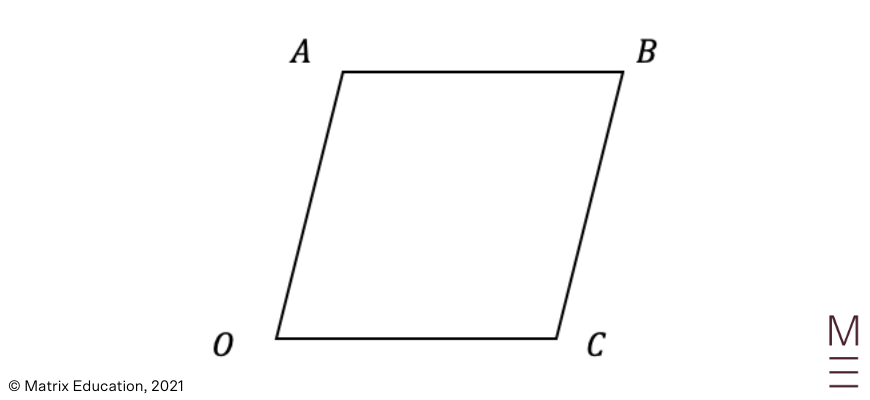### Solution:

We are required to prove that $$OB⊥AC$$. This statement is equivalent to proving that $$\overrightarrow{OB}⋅\overrightarrow{AC}=0$$.

Recall that a vector can be split into components $$i$$ and $$j$$, with $$i$$ being the x component and $$j$$ being the y component.

Hence, let $$\overrightarrow{OA}=a= \binom{a_1}{a_2}$$, and $$\overrightarrow{OC}=c= \binom{c_1}{c_2}$$.

Via vector addition and subtraction, we can also see that

$$\overrightarrow{OB}=\overrightarrow{OA}+\overrightarrow{OC}=a+c$$, and $$\overrightarrow{AC}=\overrightarrow{OC}-\overrightarrow{OA}=c-a$$.

Therefore,

 \begin{align*} \overrightarrow{OB}⋅\overrightarrow{AC} &= (a+c)⋅(c-a)\\ &= (a_1+c_1)(c_1-a_1)+(a_2+c_2)(c_2-a_2)\\ &=c_1^2-a_1^2+c_2^2-a_2^2\\ &= c_1^2+c_2^2-(a_1^2+a_2^2)\\ &= |\overrightarrow{OC}|^2-|\overrightarrow{OA}|^2\\ &= |\overrightarrow{OC}|^2-|\overrightarrow{OC}|^2 \ \ \ \text{(all sides of a rhombus have equal length)}\\ &= 0 \end{align*}

Hence, we have proven that $$OB⊥AC$$.

## Do you need extra practice with Vector Proof questions?## Concept Check Questions

1. Let $$ABCD$$ be any quadrilateral with $$P, \ Q, \ R, \ S$$ being the midpoints of the four sides. Prove (using vectors) that $$PQRS$$ is a parallelogram.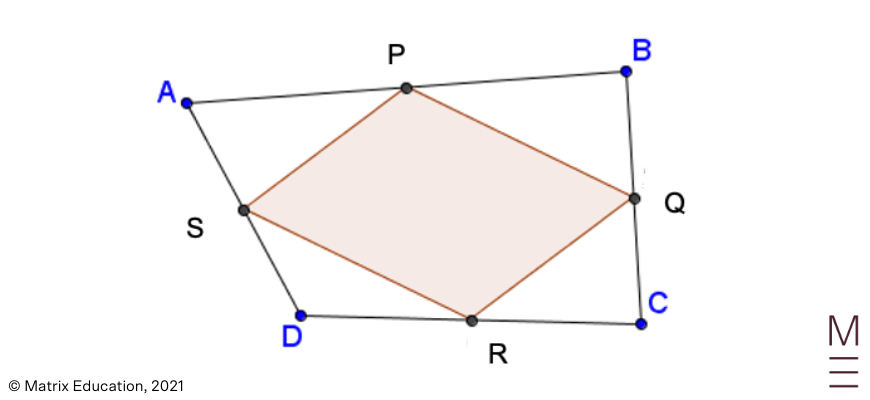2. Prove that the sum of the squares of the lengths of the diagonals of a parallelogram is equal to the sum of the squares of the lengths of the sides.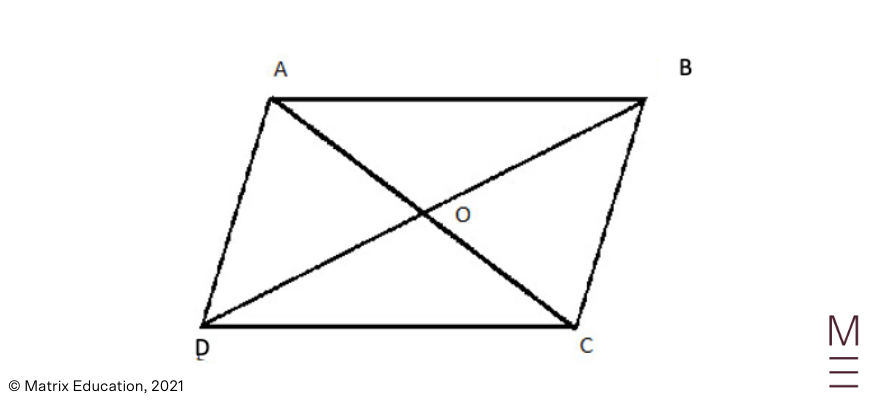3. In the following trapezium, $$AD$$ is parallel to, and five times the length of $$BC$$. It is also given that $$X$$ is the midpoint of $$AB$$ and $$Y$$ is the midpoint of $$CD$$. Use vector methods to prove that $$XY$$ is parallel to, and three times the length of $$BC$$.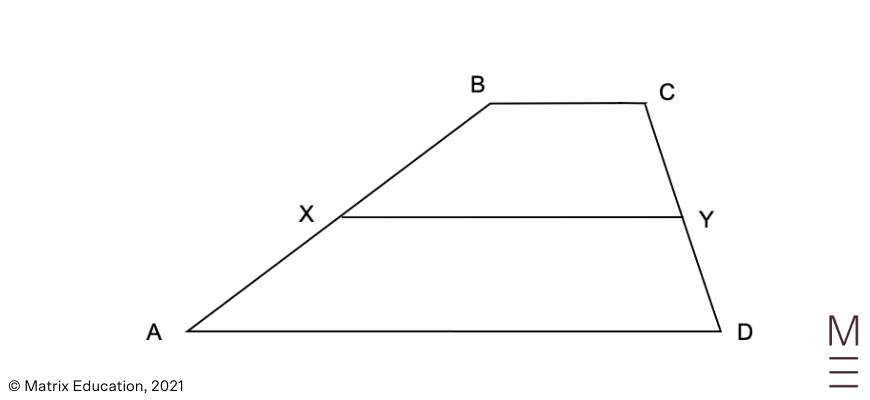## Concept Check Solutions

1. Let $$u=\overrightarrow{CR}=\overrightarrow{RD}, \ v=\overrightarrow{DS}=\overrightarrow{SA},$$ $$w=\overrightarrow{AP}=\overrightarrow{PB}, \ z=\overrightarrow{BQ}=\overrightarrow{QC}$$
We want to show that $$\overrightarrow{QR}=\overrightarrow{PS}$$ and $$\overrightarrow{RS}=\overrightarrow{QP}$$.

We begin by showing that $$\overrightarrow{QR}=\overrightarrow{PS}$$
Via vector addition, we can deduce that

\begin{align*}
\overrightarrow{QR} &= \overrightarrow{QC}+\overrightarrow{CR}=z+u\\
\overrightarrow{PS} &= \overrightarrow{PA}+\overrightarrow{AS} = -w-v\\
\end{align*}

But $$\overrightarrow{AB}+\overrightarrow{BC}=\overrightarrow{AD}+\overrightarrow{DC}$$, therefore

\begin{align*}
2w+2z&=-2v-2u\\
⇒w+z &=-v-u\\
⇒z+u &= -w-v\\
\end{align*}

which proves that $$\overrightarrow{QR}=\overrightarrow{PS}$$

Now we move on to showing that $$\overrightarrow{RS}=\overrightarrow{QP}$$.
Once again, we can deduce that

\begin{align*}
\overrightarrow{RS} &= \overrightarrow{RD}+\overrightarrow{DS}=u+v\\
\overrightarrow{QP} &= \overrightarrow{QB}+\overrightarrow{BP}=-z-w\\
\end{align*}

Since $$w+z=-v-u$$, then we can conclude that $$-z-w=u+v$$,
which proves that $$\overrightarrow{RS}=\overrightarrow{QP}$$

Therefore, $$PQRS$$ is a parallelogram.

2. We want to prove that:
$$|\overrightarrow{AC}|^2+|\overrightarrow{DB}|^2=$$$$|\overrightarrow{AB}|^2+|\overrightarrow{BC}|^2+|\overrightarrow{DC}|^2+|\overrightarrow{AD}|^2$$.

Let $$\overrightarrow{AB}=\overrightarrow{DC}=u$$, $$\overrightarrow{BC}=\overrightarrow{AD}=v$$.

\begin{align*}
\overrightarrow{DB} &=\overrightarrow{DA}+\overrightarrow{DC}=u-v\\
\end{align*}

Therefore,

 \begin{align*} LHS &= |\overrightarrow{AC}|^2+|\overrightarrow{DB}|^2\\ &= |u+v|^2+|u-v|^2\\ &=|u|^2+2|uv|+|v|^2+|u|^2-2|uv|+|v|^2\\ &= 2|u|^2+2|v|^2\\ RHS &= |\overrightarrow{AB}|^2+|\overrightarrow{BC}|^2+|\overrightarrow{DC}|^2+|\overrightarrow{AD}|^2\\ &= |u|^2+|v|^2+|u|^2+|v|^2\\ &= 2|u|^2+2|v|^2\\ &= LHS\\ \end{align*}

Hence, the statement holds.

3. Let $$u=\overrightarrow{BC}⇒\overrightarrow{AD}=5u$$. We want to prove that $$\overrightarrow{XY}=3u$$
Since $$X$$ is the midpoint of $$\overrightarrow{BA}$$, and $$Y$$ is the midpoint of $$\overrightarrow{CD}$$, then

$$\overrightarrow{BX}=\overrightarrow{XA}= \frac{1}{2}\overrightarrow{BA} \\ \overrightarrow{CY}=\overrightarrow{YD}=\frac{1}{2}\overrightarrow{CD}$$

\begin{align*}
\overrightarrow{BX}+\overrightarrow{XY} &= \overrightarrow{BC}+\overrightarrow{CY}\\
\end{align*}

By adding the above two equations together, we obtain

 \begin{align*} 2 \overrightarrow{XY}+\overrightarrow{BX}+\overrightarrow{YD} &= \overrightarrow{BC}+\overrightarrow{XA}+\overrightarrow{CY}+\overrightarrow{AD}\\ ⇒2\overrightarrow{XY} &= \overrightarrow{BC}+\overrightarrow{AD}\\ &=u+5u\\ &=6u\\ ∴\overrightarrow{XY}&=3u \ \ \ \text{, as required.}\\ \end{align*}

## Do you need that extra push to ace Maths Ext 1?

In our HSC Prep Course, our subject matter experts will revise the core topics in Maths Ext 1 through structured video lessons and provide you with plenty of practice questions and mock exams. Learn more about our HSC Prep Course now.© Matrix Education and www.matrix.edu.au, 2021. Unauthorised use and/or duplication of this material without express and written permission from this site’s author and/or owner is strictly prohibited. Excerpts and links may be used, provided that full and clear credit is given to Matrix Education and www.matrix.edu.au with appropriate and specific direction to the original content.